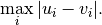# scipy.spatial.distance.chebyshev¶

scipy.spatial.distance.chebyshev(u, v)[source]

Computes the Chebyshev distance.

Computes the Chebyshev distance between two 1-D arrays u and v, which is defined asParameters : u : (N,) array_like Input vector. v : (N,) array_like Input vector. chebyshev : double The Chebyshev distance between vectors u and v.

#### Previous topic

scipy.spatial.distance.canberra

#### Next topic

scipy.spatial.distance.cityblock